Linear Momentum and Collisions

# Linear Momentum

### Learning Objectives

By the end of this section, you will be able to:

• Explain what momentum is, physically
• Calculate the momentum of a moving object

Our study of kinetic energy showed that a complete understanding of an object’s motion must include both its mass and its velocity ($K=\left(1\text{/}2\right)m{v}^{2}$). However, as powerful as this concept is, it does not include any information about the direction of the moving object’s velocity vector. We’ll now define a physical quantity that includes direction.

Like kinetic energy, this quantity includes both mass and velocity; like kinetic energy, it is a way of characterizing the “quantity of motion” of an object. It is given the name momentum (from the Latin word movimentum, meaning “movement”), and it is represented by the symbol p.

Momentum

The momentum p of an object is the product of its mass and its velocity:

$\stackrel{\to }{p}=m\stackrel{\to }{v}.$
The velocity and momentum vectors for the ball are in the same direction. The mass of the ball is about 0.5 kg, so the momentum vector is about half the length of the velocity vector because momentum is velocity time mass. (credit: modification of work by Ben Sutherland)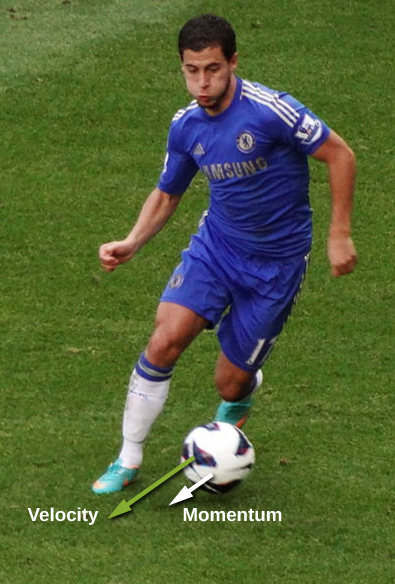As shown in (Figure), momentum is a vector quantity (since velocity is). This is one of the things that makes momentum useful and not a duplication of kinetic energy. It is perhaps most useful when determining whether an object’s motion is difficult to change ((Figure)) or easy to change ((Figure)).

This supertanker transports a huge mass of oil; as a consequence, it takes a long time for a force to change its (comparatively small) velocity. (credit: modification of work by “the_tahoe_guy”/Flickr)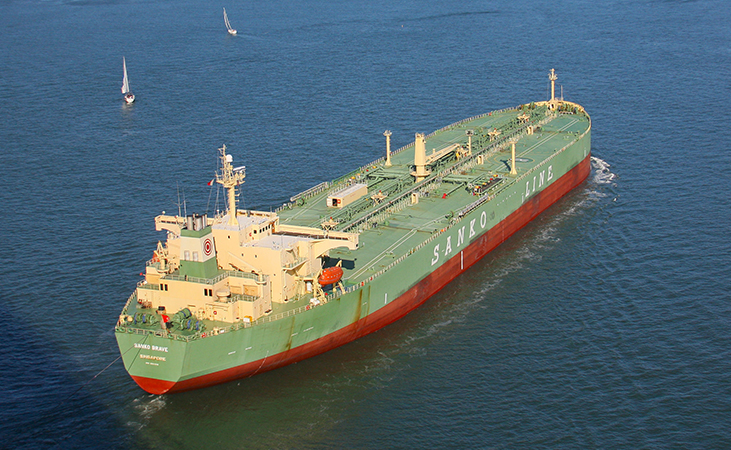Gas molecules can have very large velocities, but these velocities change nearly instantaneously when they collide with the container walls or with each other. This is primarily because their masses are so tiny.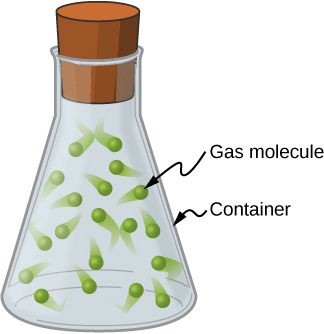Unlike kinetic energy, momentum depends equally on an object’s mass and velocity. For example, as you will learn when you study thermodynamics, the average speed of an air molecule at room temperature is approximately 500 m/s, with an average molecular mass of $6\phantom{\rule{0.2em}{0ex}}×\phantom{\rule{0.2em}{0ex}}{10}^{-25}\phantom{\rule{0.2em}{0ex}}\text{kg}$; its momentum is thus

${p}_{\text{molecule}}=\left(6\phantom{\rule{0.2em}{0ex}}×\phantom{\rule{0.2em}{0ex}}{10}^{-25}\phantom{\rule{0.2em}{0ex}}\text{kg}\right)\left(500\phantom{\rule{0.2em}{0ex}}\frac{\text{m}}{\text{s}}\right)=3\phantom{\rule{0.2em}{0ex}}×\phantom{\rule{0.2em}{0ex}}{10}^{-22}\phantom{\rule{0.2em}{0ex}}\frac{\text{kg}·\text{m}}{\text{s}}.$

For comparison, a typical automobile might have a speed of only 15 m/s, but a mass of 1400 kg, giving it a momentum of

${p}_{\text{car}}=\left(1400\phantom{\rule{0.2em}{0ex}}\text{kg}\right)\left(15\phantom{\rule{0.2em}{0ex}}\frac{\text{m}}{\text{s}}\right)=21,000\phantom{\rule{0.2em}{0ex}}\frac{\text{kg}·\text{m}}{\text{s}}.$

These momenta are different by 27 orders of magnitude, or a factor of a billion billion billion!

### Summary

• The motion of an object depends on its mass as well as its velocity. Momentum is a concept that describes this. It is a useful and powerful concept, both computationally and theoretically. The SI unit for momentum is kg$·$ m/s.

### Conceptual Questions

An object that has a small mass and an object that has a large mass have the same momentum. Which object has the largest kinetic energy?

Since $K={p}^{2}\text{/}2m$, then if the momentum is fixed, the object with smaller mass has more kinetic energy.

An object that has a small mass and an object that has a large mass have the same kinetic energy. Which mass has the largest momentum?

### Problems

An elephant and a hunter are having a confrontation.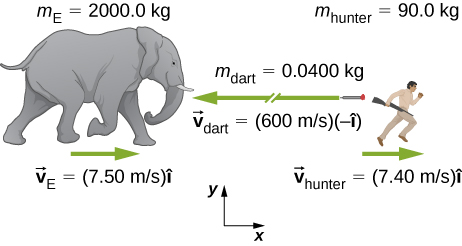1. Calculate the momentum of the 2000.0-kg elephant charging the hunter at a speed of 7.50 m/s.
2. Calculate the ratio of the elephant’s momentum to the momentum of a 0.0400-kg tranquilizer dart fired at a speed of 600 m/s.
3. What is the momentum of the 90.0-kg hunter running at 7.40 m/s after missing the elephant?

A skater of mass 40 kg is carrying a box of mass 5 kg. The skater has a speed of 5 m/s with respect to the floor and is gliding without any friction on a smooth surface.

1. Find the momentum of the box with respect to the floor.
2. Find the momentum of the box with respect to the floor after she puts the box down on the frictionless skating surface.

a. magnitude: $25\phantom{\rule{0.2em}{0ex}}\text{kg}·\text{m/s;}$ b. same as a.

A car of mass 2000 kg is moving with a constant velocity of 10 m/s due east. What is the momentum of the car?

The mass of Earth is $5.97\phantom{\rule{0.2em}{0ex}}×\phantom{\rule{0.2em}{0ex}}{10}^{24}\phantom{\rule{0.2em}{0ex}}\text{kg}$ and its orbital radius is an average of $1.50\phantom{\rule{0.2em}{0ex}}×\phantom{\rule{0.2em}{0ex}}{10}^{11}\phantom{\rule{0.2em}{0ex}}\text{m}$. Calculate the magnitude of its average linear momentum.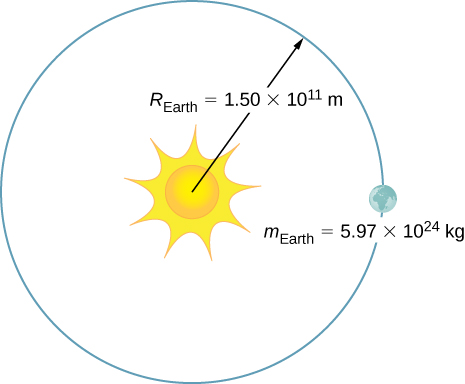$1.78\phantom{\rule{0.2em}{0ex}}×\phantom{\rule{0.2em}{0ex}}{10}^{29}\phantom{\rule{0.2em}{0ex}}\text{kg}·\text{m/s}$

If a rainstorm drops 1 cm of rain over an area of 10 km2 in the period of 1 hour, what is the momentum of the rain that falls in one second? Assume the terminal velocity of a raindrop is 10 m/s.

What is the average momentum of an avalanche that moves a 40-cm-thick layer of snow over an area of 100 m by 500 m over a distance of 1 km down a hill in 5.5 s? Assume a density of 350 kg/m3 for the snow.

$1.3\phantom{\rule{0.2em}{0ex}}×\phantom{\rule{0.2em}{0ex}}{10}^{9}\phantom{\rule{0.2em}{0ex}}\text{kg}·\text{m/s}$

What is the average momentum of a 70.0-kg sprinter who runs the 100-m dash in 9.65 s?

### Glossary

momentum
measure of the quantity of motion that an object has; it takes into account both how fast the object is moving, and its mass; specifically, it is the product of mass and velocity; it is a vector quantity